# 1.2   Built-in Types of Data

A data type is a set of values and a set of operations defined on them. For example, we are familiar with numbers and with operations defined on them such as addition and multiplication. There are eight different built-in types of data in Java, mostly different kinds of numbers. We use the system type for strings of characters so frequently that we also consider it here.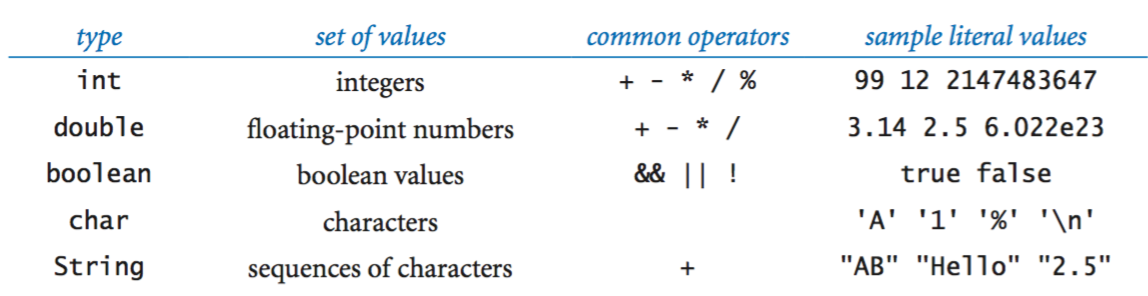Terminology. We use the following code fragment to introduce some terminology:

 int a, b, c; a = 1234; b = 99; c = a + b; 

The first line is a declaration statement that declares the names of three variables using the identifiers a, b, and c and their type to be int. The next three lines are assignment statements that change the values of the variables, using the literals 1234 and 99, and the expression a + b, with the end result that c has the value 1333.

Characters and strings. A char is an alphanumeric character or symbol, like the ones that you type. We usually do not perform any operations on characters other than assigning values to variables. A String is a sequence of characters. The most common operation that we perform on strings is known as concatenation: given two strings, chain them together to make a new string. For example, consider the following Java program fragment:

 String a, b, c; a = "Hello,"; b = " Bob"; c = a + b; 

The first statement declares three variables to be of type String. The next three statements assign values to them, with the end result that c has the value "Hello, Bob". Using string concatenation, Ruler.java prints the relative lengths of the subdivisions on a ruler.

Integers. An int is an integer (whole number) between −231 and 231 − 1   (−2,147,483,648 to 2,147,483,647). We use ints frequently not just because they occur frequently in the real world, but also they naturally arise when expressing algorithms. Standard arithmetic operators for addition, multiplication, and division, for integers are built into Java, as illustrated in IntOps.java and the following table: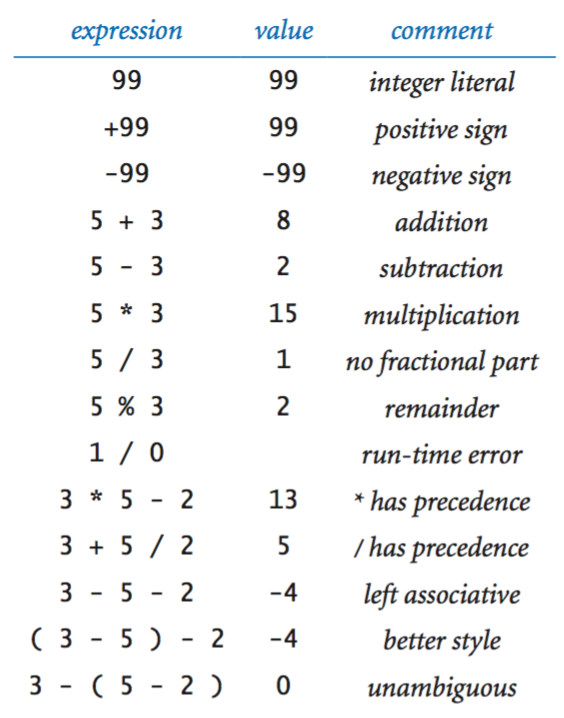Floating-point numbers. The double type is for representing floating-point numbers, e.g., for use in scientific applications. The internal representation is like scientific notation, so that we can compute with real numbers in a huge range. We can specify a floating point number using either a string of digits with a decimal point, e.g., 3.14159 for a six-digit approximation to the mathematical constant pi, or with a notation like scientific notation, e.g., 6.022E23 for Avogadro's constant 6.022 × 1023. Standard arithmetic operators for addition, multiplication, and division, for doubles are built in to Java, as illustrated in DoubleOps.java and the following table: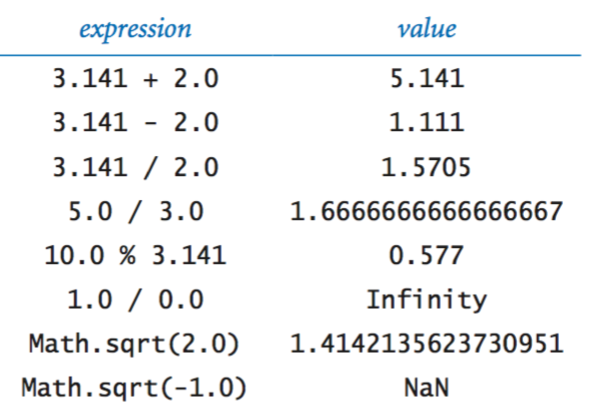Quadratic.java shows the use of doubles in computing the two roots of a quadratic equation using the quadratic formula.

Booleans. The boolean type has just two values: true or false. The apparent simplicity is deceiving—booleans lie at the foundation of computer science. The most important operators defined for the boolean are for and, or, and not.

• anda && b is true if both a and b are true, and false otherwise.
• ora || b is true if either a or b is true (or both are true), and false otherwise
• not!a is true if a is false, and false otherwise.

Although these definitions are intuitive and easy to understand, it is worthwhile to fully specify each possibility for each operation in a truth table.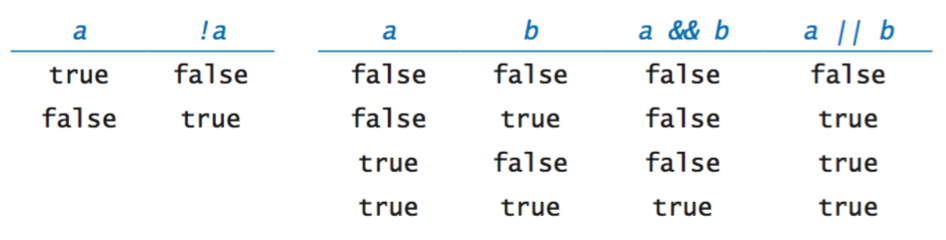Comparisons. The comparison operators are mixed-type operations that take operands of one type (e.g., int or double) and produce a result of type boolean. These operations play a critical role in the process of developing more sophisticated programs.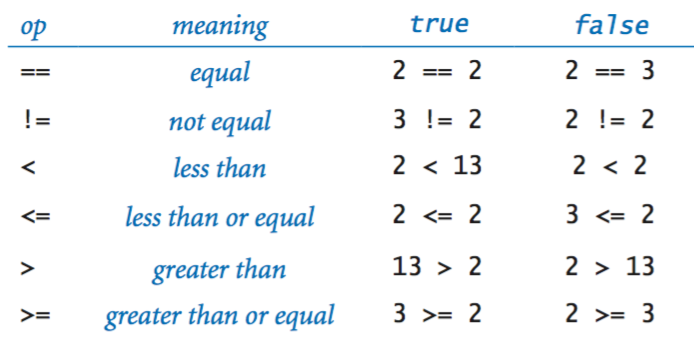LeapYear.java tests whether an integer corresponds to a leap year in the Gregorian calendar.

## Library methods and APIs.

Many programming tasks involve using Java library methods in addition to the built-in operators. An application programming interface is a table summarizing the methods in a library.
• Printing strings to the terminal window.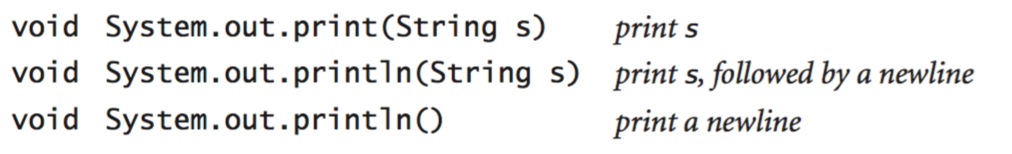• Converting strings to primitive types.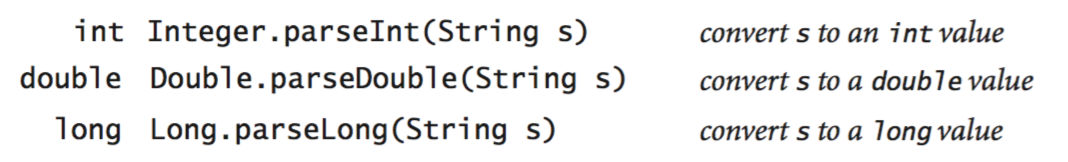• Mathematical functions.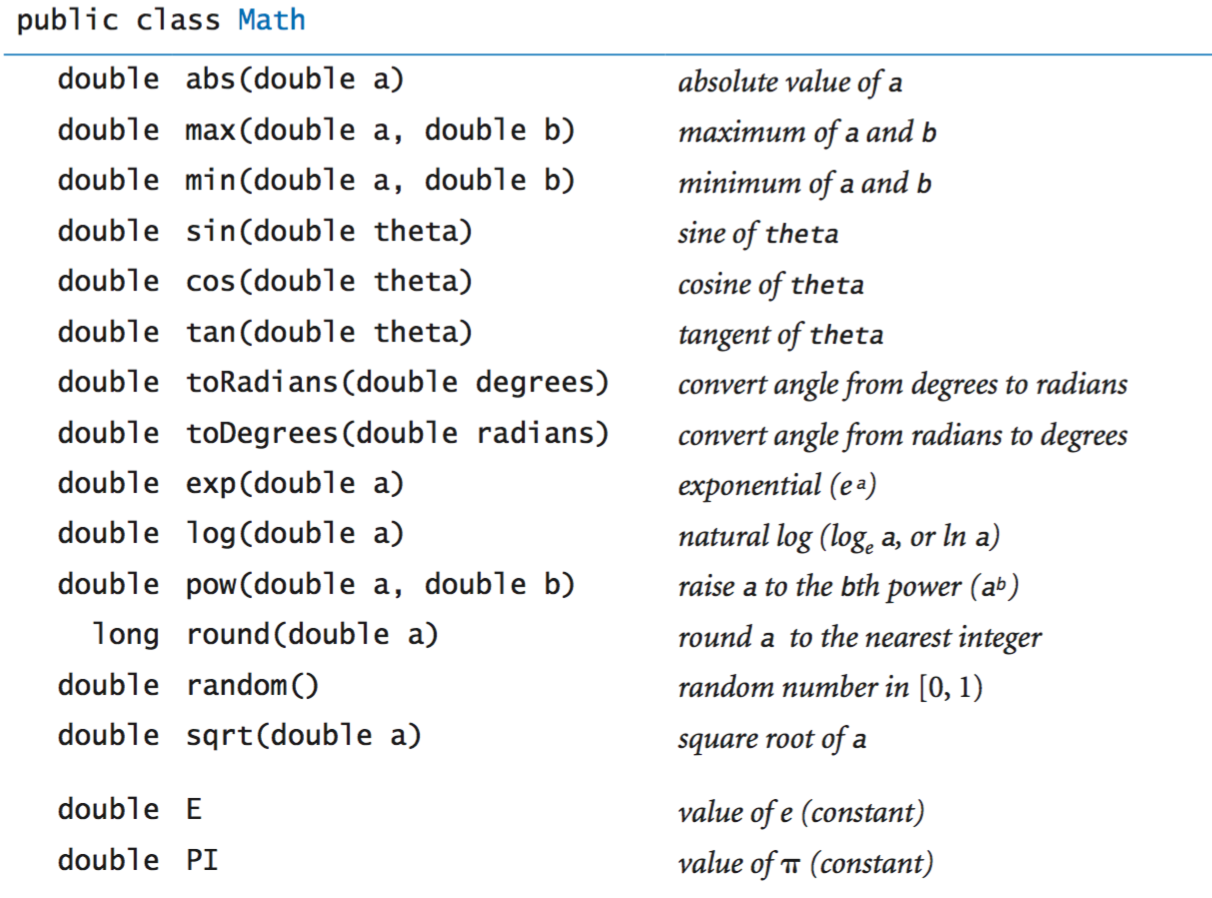You can call a method by typing its name followed by arguments, enclosed in parentheses and separated by commas. Here are some examples: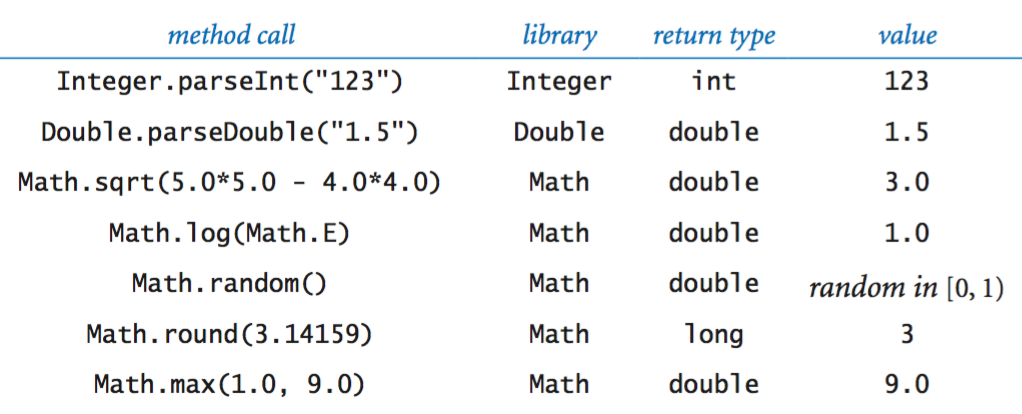We often find ourselves converting data from one type to another using one of the following approaches.

## Type conversion.

We often find ourselves converting data from one type to another using one of the following approaches.
• Explicit type conversion. Call methods such as Math.round(), Integer.parseInt(), and Double.parseDouble().
• Automatic type conversion. For primitive numeric types, the system automatically performs type conversion when we use a value whose type has a larger range of values than expected.
• Explicit casts. Java also has some built-in type conversion methods for primitive types that you can use when you are aware that you might lose information, but you have to make your intention using something called a cast. RandomInt.java reads an integer command-line argument n and prints a "random" integer between 0 and n−1.
• Automatic conversions for strings. The built-in type String obeys special rules. One of these special rules is that you can easily convert any type of data to a String by using the + operator.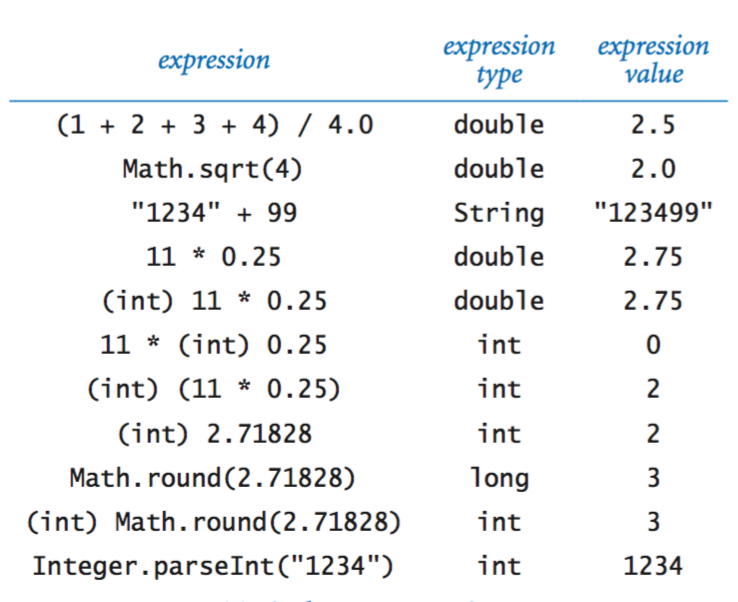#### Exercises

1. Suppose that a and b are int values. What does the following sequence of statements do?
 int t = a; b = t; a = b; 

Solution: sets a, b, and t equal to the original value of a.

2. Suppose that a and b are int values. Simplify the following expression: (!(a < b) && !(a > b))

Solution: (a == b)

3. The exclusive or operator ^ for boolean operands is defined to be true if they are different, false if they are the same. Give a truth table for this function.
4. Why does 10/3 give 3 and not 3.33333333?

Solution: Since both 10 and 3 are integer literals, Java sees no need for type conversion and uses integer division. You should write 10.0/3.0 if you mean the numbers to be double literals. If you write 10/3.0 or 10.0/3, Java does implicit conversion to get the same result.

5. What do each of the following print?
1. System.out.println(2 + "bc"); prints: 2bc
2. System.out.println(2 + 3 + "bc"); prints: 5bc
3. System.out.println((2+3) + "bc"); prints: 5bc
4. System.out.println("bc" + (2+3)); prints: bc5
5. System.out.println("bc" + 2 + 3); prints: bc23

Explain each outcome.

6. Explain how to use Quadratic.java to find the square root of a number.

Solution: to find the square root of c, find the roots of x^2 + 0x - c.

7. A physics student gets unexpected results when using the code
double force = G * mass1 * mass2 / r * r;

to compute values according to the formula F = Gm1m2 / r2. Explain the problem and correct the code.

Solution: It divides by r, then multiplies by r (instead of dividing by r *r). Use parentheses:

double force = G * mass1 * mass2 / (r * r);

8. Write a program Distance.java that takes two integer command-line arguments x and y and prints the Euclidean distance from the point (x, y) to the origin (0, 0).
9. Write a program SumOfTwoDice.java that prints the sum of two random integers between 1 and 6 (such as you might get when rolling dice).
10. Write a program SumOfSines.java that takes a double command-line argument t (in degrees) and prints the value of sin(2t) + sin(3t).
11. Write a program SpringSeason.java that takes two int values m and d from the command line and prints true if day d of month m is between March 20 (m = 3, d =20) and June 20 (m = 6, d = 20), false otherwise.

#### Creative Exercises

1. Wind chill. Given the temperature t (in Fahrenheit) and the wind speed v (in miles per hour), the National Weather Service defines the wind chill to be:
w = 35.74 + 0.6215 t + (0.4275 t - 35.75) v0.16
Write a program WindChill.java that takes two double command-line arguments t and v and prints the wind chill. Use Math.pow(a, b) to compute ab. Note: the formula is not valid if t is larger than 50 in absolute value or if v is larger than 120 or less than 3 (you may assume that the values you get are in that range).
2. Polar coordinates. Write a program CartesianToPolar.java that converts from Cartesian to polar coordinates. Your program should take two real numbers x and y on the command line and print the polar coordinates r and θ. Use the Java method Math.atan2(y, x), which computes the arctangent value of y/x that is in the range from -π to π.
3. Day of the week. Write a program DayOfWeek.java that takes a date as input and prints the day of the week that date falls on. Your program should take three command-line arguments: m (month), d (day), and y (year). For m use 1 for January, 2 for February, and so forth. For output print 0 for Sunday, 1 for Monday, 2 for Tuesday, and so forth. Use the following formulas, for the Gregorian calendar (where / denotes integer division):
y0 = y − (14 − m) / 12
x = y0 + y0 / 4 − y0 / 100 + y0 / 400
m0 = m + 12 × ((14 − m) / 12) − 2
d0 = (d + x + 31m0 / 12) mod 7
For example, on which day of the week did February 14, 2000 fall?
 y0 = 2000 - 0 = 1999 x = 1999 + 1999/4 - 1999/100 + 1999/400 = 2483 m0 = 2 + 12*1 - 2 = 12 d0 = (13 + 2483 + (31*12) / 12) mod 7 = 2528 mod 7 = 1 (Monday) 
4. Uniform random numbers. Write a program Stats5.java that prints five uniform random values between 0 and 1, their average value, and their minimum and maximum value. Use Math.random(), Math.min(), and Math.max().
5. Three-sort. Write a program ThreeSort.java that takes three int values from the command line and prints them in ascending order. Use Math.min() and Math.max().
6. Dragon curves.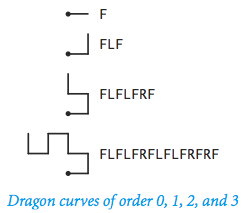Write a program Dragon.java to print the instructions for drawing the dragon curves of order 0 through 5. The instructions are strings of the characters F, L, and R, where F means "draw line while moving 1 unit forward", L means "turn left", and R means turn right. A dragon curve of order n is formed when you fold a strip of paper in half n times, then unfold to right angles. The key to solving this problem is to note that a curve of order n is a curve of order n−1 followed by an L followed by a curve of order n−1 traversed in reverse order, and then to figure out a similar description of the reverse curve.

#### Web Exercises

1. Write a program Swap.java that takes two integer command-line arguments a and b and swaps their values using the swapping idiom described on p. 17. After each assignment statement, use System.out.println() to print a trace of the variables.
2. What does the following statement do where grade is a variable of type int?
 boolean isA = (90 <= grade <= 100); 

Solution: Syntax error since <= is a binary operator. You can rewrite the expression as (90 <= grade && grade <= 100).

3. RGB to YIQ color converter. Write a program RGBtoYIQ.javathat takes an RGB color (three integers between 0 and 255) and transforms it to a YIQ color (three different real numbers Y, I, and Q, with 0 ≤ Y ≤ 1, –0.5957 ≤ I ≤ 0.5957, and –0.5226 ≤ Q ≤ 0.5226). Write a program YIQtoRGB.java that applies the inverse transformation.
4. CMYK to RGB color matching. Write a program CMYKtoRGB that reads in four command line inputs C, M, Y, and K between 0 and 1, and prints the corresponding RGB parameters. Devise the appropriate formula by "inverting" the RGB to CMYK conversion formula.
5. What does the following code fragment print?
 double x = (double) (3/5); System.out.println(x); 

Solution: It prints 0.0 since the integer division is done before the cast.

6. Why doesn't the following program print 4294967296 = 2^32?
 int x = 65536; long y = x * x; System.out.println(y); 
Solution: The product of the two int values is computed as an int, and then automatically converted to a long. But 65536 * 65536 = 2^32 overflows a 32 bit int before it gets converted.
7. What is the value of (Math.sqrt(2) * Math.sqrt(2) == 2)?
8. Write a program DivideByZero.java to see what happens when you divide an int or double by zero.

Solution:

• (17 / 0) and (17 % 0) result in a division by zero exception;
• (17.0 / 0.0) results in a value Infinity;
• (17.0 % 0.0) results in a value NaN that stands for "not a number."
9. Guess the biggest number. Consider the following game. Alice writes down two integers between 0 and 100 on two cards. Bob gets to select one of the two cards and see its value. After looking at the value, Bob commits to one of the two cards. If he chooses a card with the largest value, he wins; otherwise he loses. Devise a strategy (and corresponding computer program) for Bob so that he guarantees to win strictly more than half the time.
10. Fibonacci word. Write a program FibonacciWord.java that prints the Fibonacci word of order 0 through 10. f(0) = "a", f(1) = "b", f(2) = "ba", f(3) = "bab", f(4) = "babba", and in general f(n) = f(n-1) followed by f(n-2). Use string concatenation.
11. Standard deviation. Modify Exercise 1.2.30 so that it prints the sample standard deviation in addition to the average.
12. Write a program that reads in three parameters and prints true if all three are equal, and false otherwise.
13. What happens if you compile LeapYear.java and execute it with
1. java LeapYear
2. java LeapYear 1975.5
3. java LeapYear -1975
4. java LeapYear 1975 1976 1977
14. What does the compiler do if you try to write the following expression:
 int a = 27 * "three"; 
15. What does the compiler do if you try to write the following expression:
 double x; System.out.println(x); 

Solution: The compiler complains that the variable x might not have been initialized. Variables within main are not automatically initialized.

16. What does the following code fragment print.
 int threeInt = 3; int fourInt = 4; double threeDouble = 3.0; double fourDouble = 4.0; System.out.println(threeInt / fourInt); System.out.println(threeInt / fourDouble); System.out.println(threeDouble / fourInt); System.out.println(threeDouble / fourDouble); 
17. Write a program that takes four real-valued command line parameters x1, y1, x2, and y2 and prints the Euclidean distance between the points (x1, y1) and (x2, y2). Use Math.sqrt().

18. Write a program Ordered.java that reads in three integer command line arguments, x, y, and z. Create a boolean variable b that is true if the three values are either in ascending or in descending order, and false otherwise. Print the variable b.
19. Write a program Divisibility.java that reads in two command line inputs and prints true if both are divisible by 7, and false otherwise.
20. Area of a triangle. Write a program TriangleArea.java that takes three command line inputs a, b, and c, representing the side lengths of a triangle, and prints the area of the triangle using Heron's formula: area = sqrt(s(s-a)(s-b)(s-c)), where s = (a + b + c) / 2.
21. Equatorial to horizontal coordinates. The equatorial coordinate system is widely used by astronomers to indicate the position of a star on the celestial sphere. The position is specified by its declination δ, its hour angle H, and its latitude φ. The horizontal coordinate system (a.k.a. Alt/Az coordinate system) is useful for determining the setting/rising time of celestial objects. The position is specified by its altitude (vertical angle from the horizon) and and its azimuth (horizontal angle). Given a star's position using equatorial coordinates, find its position in horizontal coordinates using the formulas below.
 Altitude = asin (sin φ sin δ + cos φ cos δ cos H) Azimuth = acos ((cos φ sin δ - sin φ cos δ cos H) / cos (Altitude) ) 
22. Body mass index. The body mass index (BMI) is the ratio of the weight of a person (in kilograms) to the square of the height (in meters). Write a program BMI.java that takes two command-line arguments, weight and height, and prints the BMI.
23. Temperature conversion. What is wrong with the following code fragment to convert from Fahrenheit (F) to Celsius (C)?
 double C = (F - 32) * (5 / 9); 
24. Exponentiation. What is wrong with the following code fragment to compute a2, where a is of type double?
 double b = a^2; 

Solution: In Java, ^ does not mean exponentiation (it's the exclusive or function from logic). Use a*a instead. To compute ax, use Math.pow(a, x). Note that Math.pow() returns a double so that you would need an explicit cast if a and b in the above example were integers.

25. What of the following statements is legal?
 boolean b = 1; boolean b = true; boolean b = "true"; boolean b = True; 

Solution: Only the second one.

26. Barring overflow, give a code fragment to compute the maximum of two integers a and b without using Math.max() or if.
 int max = (a + b + Math.abs(a - b)) / 2; 
27. Discriminant of cubic polynomial. Given the coefficients b, c, and d of the cubic polynomial x^3 + bx^2 + cx + d, write an expression to compute the discriminant b^2c^2 - 4c^3 - 4b^3d - 27d^2 + 18bcd.
28. Barycenter. In a two-body system, the barycenter is the center of gravity about which the two celestial bodies orbit each other. Given the masses m1 and m2 of two bodies, and the shortest distance a between the two bodies, write a program to compute the distance from the center of the first (more massive) body to the barycenter: r1 = a m2 / (m1 + m2).

Here are a few examples. Masses are in earth-mass units, distances are in kilometers.

Earth-moon: m1 = 1, m2 = .0123, a = 384,000, r1 = 4,670, R1 = 6,380.

Pluto-Charon: m1 = .0021, m2 = .000254, a = 19,600, r1 = 2,110, R1 = 1,150.

Sun-Earth: m1 = 333,000, m2 = 1, a = 150,000,000, r1 = 449, R1 = 696,000.

Note that if r1 is less than the radius of the first body R1, then the barycenter lies within the first body.

29. Poison parentheses. Find a legal Java expression that leads to a compile-time error when you add parentheses around a subexpression to to document the order of evaluation that would take place in their absence.

Solution: The literal value 2147483648 (2^31) is permitted only as an operand of the unary minus operator, i.e., -2147483648. Enclosing it in parentheses, i.e., -(2147483648), leads to a compile-time error. Similar ideas with the literal value 9223372036854775808L (2^63).

30. Car loan payments. Write a program CarLoan.java that reads in three command-line arguments P, Y, and R and calculates the monthly payments you would have to make over Y years to pay off a P dollar loan at R per cent interest compounded monthly. The formula is The formula is
  P r payment = ---------------, where n = 12 * Y, r = (R / 100) / 12 1 - (1 + r)^(-n) 

Caveat: in Chapter 9, we will consider more accurate ways to compute this quantity, so before you go off to run an online bank, be sure to learn about roundoff error.

31. Write a program Trig.java to illustrate various trigonometric functions in the Math library, such as Math.sin(), Math.cos(), and Math.toRadians().